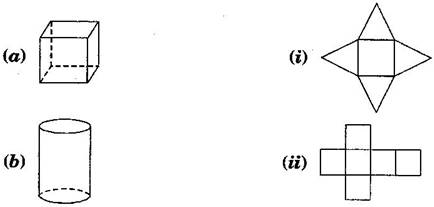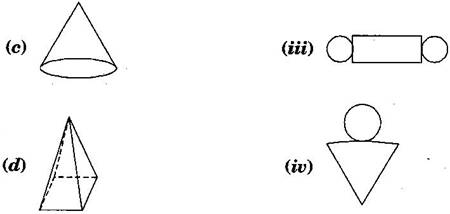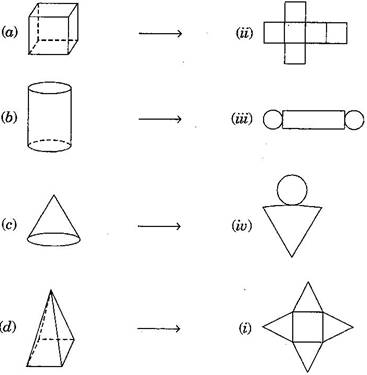### Visualising Solid Shapes - Solutions

CBSE Class –VII Mathematics

NCERT Solutions
Chapter 15 Visualising Solid Shapes (Ex. 15.1)

Question 1. Identify the nets which can be used to make cubes (cut out copies of the nets and try it)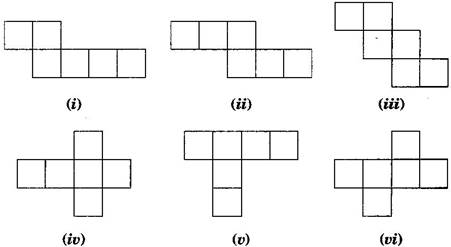Answer : Cube’s nets are (ii), (iii), (iv) and (vi).

Question 2. Dice are cubes with dots on each face. Opposite faces of a die always have a total of seven dots on them.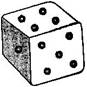Here are two nets to make dice (cubes); the numbers inserted in each square indicate the number of dots in that box.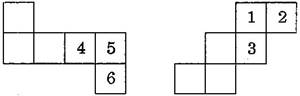Insert suitable numbers in the blanks, remembering that the number on the opposite faces should total to 7.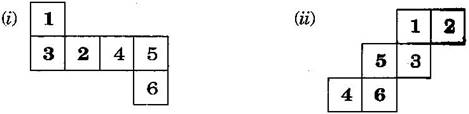Question 3. Can this be a net for a die? Explain your answer.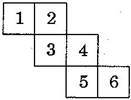Answer : No, this cannot be a net for a die.

Because one pair of opposite faces will have 1 and 4 on them and another pair of opposite faces will have 3 and 6 on them whose total is not equal to 7.

Question 4. Here is an incomplete net for making a cube. Complete it in at least two different ways. Remember that a cube has six faces. How many faces are there in the net here? (Give two separate diagrams. If you like, you may use a squared sheet for easy manipulation.)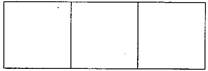Answer : There three faces are given: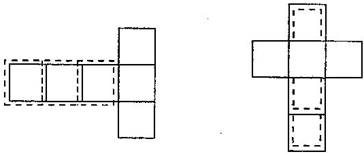Question 5. Match the nets with appropriate solids: